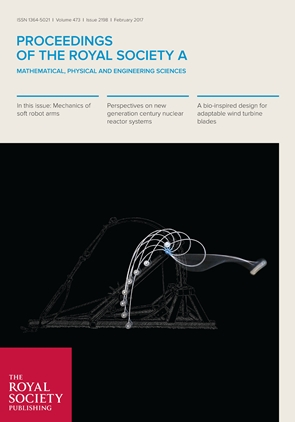Professor of Solid and Structural Mechanics

# The elastica compass and the elastica catapultAn elastic rod is clamped at one end and has a dead load attached to the other (free) end. The rod is then slowly rotated using the clamp. When the load is smaller than the buckling value, the rod describes a continuous set of quasi-static forms and its end traces a (smooth, convex and simple) closed curve, which would be a circle if the rod were rigid. The closed curve is analytically determined through the integration of the Eulers elastica, so that for sufficiently small loads the mechanical system behaves as an elastica compass. For loads higher than that of buckling, the elastica reaches a configuration from which a snap-back instability occurs, realizing a sort of elastica catapult. The whole quasi-static evolution leading to the critical configuration for snapping is calculated through the elastica and the subsequent dynamic motion simulated using two numerical procedures, one ad hoc developed and another based on a finite element scheme. The theoretical results are then validated on a specially designed and built apparatus. An obvious application of the present model would be in the development of soft robotic limbs, but the results are also of interest for the optimization analysis in pole vaulting.

Related papers: